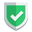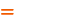我们相信：世界是美好的，你是我也是。平行空间的世界里面，不同版本的生活也在继续...

## 数据表及结构

``````DROP TABLE IF EXISTS `test_table`;
CREATE TABLE `test_table` (
`Id` int(11) NOT NULL AUTO_INCREMENT,
`name` varchar(255) DEFAULT NULL,
`comment` varchar(255) DEFAULT NULL,
PRIMARY KEY (`Id`)
) ENGINE=MyISAM AUTO_INCREMENT=4 DEFAULT CHARSET=utf8mb4;
INSERT INTO `test_table` VALUES (1,'NULL','NULL string'),(2,NULL,'real NULL'),(3,'','just blank');``````

## `thinkphp`准备工作

`thinkphp@5.0.24完整版`下载地址：

`application/database.php`，配置数据库信息，一般来说，就是修改`database``password`信息：

`application/database.php`，开启`app_debug``app_trace`

``````return [
// 应用调试模式
'app_debug'              => true,
// 应用Trace
'app_trace'              => true,
//...
}``````

## 基础类引用

`application/index/controller/Index.php`

``use think\Db;``

## 正确答案

``SELECT * FROM `test_table` WHERE `name` IS NULL``

``````Db::table('test_table')->where('name','null')->select();
Db::table('test_table')->where('name',null)->select();
Db::table('test_table')->where('name is null')->select();
Db::table('test_table')->where('name','exp','is null')->select();
Db::table('test_table')->where(["name"=>null])->select();
Db::table('test_table')->where(["name"=>`null`])->select();
Db::table('test_table')->where(["name"=>`is null`])->select();``````

``````\$p["name"]=['exp',new DB\Expression('IS NULL')];
Db::table('test_table')->where(\$p)->select();``````

``Db::table('test_table')->where('name','not null')->select();``

## 错误答案

### 错误答案一

``````\$params = [];
\$params["name"] = "is null";
\$row = Db::table('test_table')->where(\$params)->select();``````

``SELECT * FROM `test_table` WHERE `name` = 'is null'``

### 错误答案二

``````\$params=[];
\$params["name"]=[`eq`,`null`];
\$row2=Db::table('test_table')->where(\$params)->select();``````

``````\$params = [];
\$params["name"] = ['eq', 'NULL'];
\$row3 = Db::table('test_table')->where(\$params)->select();``````

``SELECT * FROM `test_table` WHERE `name` = 'NULL'``

### 错误答案三

``````\$params = [];
\$params["name"]=["EXP","IS NULL"];
//\$params["name"]=["EXP","NULL"];
\$row3 = Db::table('test_table')->where(\$params)->select();``````

`exp`这种方式，是`thinkphp`官方社区特别推荐的。但是，所列出的方式，在最新版的`thinkphp@5.0.24`中，却直接报错：

``查询表达式错误:EXP``

``````\$p=[];
\$p["name"]=['exp',new DB\Expression('IS NULL')];
\$row9 = Db::table('test_table')->where(\$p)->select();``````

## 相关链接

`thinkphp5`官方文档里面的相关描述文字，如下：

## 总结如果本文对您有帮助，或者节约了您的时间，欢迎打赏瓶饮料，建立下友谊关系。本博客不欢迎：各种镜像采集行为。请尊重原创文章内容，转载请保留作者链接。【福利】 腾讯云最新爆款活动！1核2G云服务器首年50元！【源码】本文代码片段及相关软件，请点此获取更多信息【绝密】秘籍文章入口，仅传授于有缘之人php    mysql